Theory and Modern Applications

# Positive solutions of a singular semipositone boundary value problems for fourth-order coupled difference equations

## Abstract

In this article, we study the boundary value problems for the fourth-order nonlinear coupled difference equations

$\left\{\begin{array}{c}{\mathrm{△}}^{4}u\left(i-2\right)={f}_{1}\left(i,v\left(i\right)\right)+{e}_{1}\left(i\right),\phantom{\rule{1em}{0ex}}i\in \left[2,T+2\right],\hfill \\ {\mathrm{△}}^{4}v\left(i-2\right)={f}_{2}\left(i,u\left(i\right)\right)+{e}_{2}\left(i\right),\phantom{\rule{1em}{0ex}}i\in \left[2,T+2\right],\hfill \\ u\left(0\right)=u\left(1\right)=v\left(1\right)=v\left(0\right)=0,\hfill \\ u\left(T+3\right)=u\left(T+4\right)=v\left(T+4\right)=v\left(T+3\right)=0.\hfill \end{array}$

Throughout our nonlinearity may be singular. We establish the existence of positive solutions for the fourth-order coupled systems. The proof relies on Schauder’s fixed point theorem.

MSC:39A11.

## Introduction

In this article, we consider the following boundary value problems of difference equations

$\left\{\begin{array}{c}{\mathrm{△}}^{4}u\left(i-2\right)={f}_{1}\left(i,v\left(i\right)\right)+{e}_{1}\left(i\right),\phantom{\rule{1em}{0ex}}i\in \left[2,T+2\right],\hfill \\ {\mathrm{△}}^{4}v\left(i-2\right)={f}_{2}\left(i,u\left(i\right)\right)+{e}_{2}\left(i\right),\phantom{\rule{1em}{0ex}}i\in \left[2,T+2\right],\hfill \\ u\left(0\right)=u\left(1\right)=v\left(1\right)=v\left(0\right)=0,\hfill \\ u\left(T+3\right)=u\left(T+4\right)=v\left(T+4\right)=v\left(T+3\right)=0,\hfill \end{array}$
(1)

here $\left[2,T+2\right]=\left\{2,3,\dots ,T+2\right\}$ and $u,v,{e}_{j}:\left[0,T+4\right]\to R$, ${f}_{j}:\left[0,T+4\right]×R\to {R}^{+}$, $j=1,2$. We will let $\left[a,b\right]$ denote the discrete integer set $\left[a,b\right]=\left\{a,a+1,\dots ,b\right\}$, $C\left(\left[a,b\right]\right)$ denote set of continuous function on $\left[a,b\right]$ (discrete topology) with norm $\parallel \cdot \parallel ={max}_{k\in \left[a,b\right]}|\cdot |$.

Recently, many literature on the boundary value of difference equations have appeared. We refer the reader to  and the references therein, which include work on Agarwal, Eloe, Erber, O’Regan, Henderson, Merdivenci, Yu, Ma et al., concerning the existence of positive solutions and the corresponding eigenvalue problems. Recently, the existence of positive solutions of fourth-order discrete boundary value problems have been studied by several authors, for examples, see  and the references therein.

The fourth-order boundary value problems of ordinary value problems have important application in various branches of pure and applied science. As in entrepreneurial network evolution studies, the research paradigm of ‘U related to V’ was always applied, while U or V was the measurement of the attitudes of the entrepreneurs who answered the questionnaire. According to cognitive psychology studies [19, 20], questionnaire shows the change of attitude, which is the fourth-order dependent of original signal. So whether this paradigm will lead to meaningful causal outcome, which is basically depended on difference equations.

The fourth-order boundary value problems of ordinary value problems have important application in various branches of pure and applied science. They arise in the mathematical modeling of viscoelastic and inelastic flows, deformation of beams and plate deflection theory . For examples, the deformations of an elastic beam can be described by the boundary value problems of the fourth-order ordinary differential equations. There have been extensive studies on fourth-order boundary value problems with diverse boundary conditions via many methods, for example  and the references therein.

The remaining part of the article is organized as follows. In Section “Preliminaries”, some preliminary results will be given. In the remaining sections, by employing a basic application of Schauder’s fixed point theorem, we state and prove the existence results for (1). Our view point sheds some new light on problems with weak force potentials and prove that in some situations weak singularities may stimulate the existence of positive solutions.

## Preliminaries

In this section, we state the preliminary information that we need to prove the main results. From [4, 5], we have the following lemma.

Lemma 1 $x\left(i\right)$ is a solution of equation

$\left\{\begin{array}{c}{\mathrm{△}}^{4}x\left(i-2\right)=h\left(i\right),\phantom{\rule{1em}{0ex}}i\in \left[2,T+2\right],\hfill \\ x\left(0\right)=x\left(1\right)=x\left(T+3\right)=x\left(T+4\right)=0\hfill \end{array}$
(2)

if only and if

$x\left(i\right)=\sum _{j=2}^{T+2}G\left(i,j\right)h\left(j\right),\phantom{\rule{1em}{0ex}}i\in \left[0,T+4\right],$
(3)

where

$G\left(i,j\right)=\left\{\begin{array}{cc}\frac{{\left(T+4-i\right)}^{2}{\left(j-1\right)}^{2}}{2}\left(\frac{i}{{\left(T+3\right)}^{2}}-\frac{\left(T+4+2i\right)\left(j-1\right)}{3{\left(T+4\right)}^{3}}\right),\hfill & 2\le j\le i+1,\hfill \\ \frac{{i}^{2}{\left(T+4-j\right)}^{2}}{6}\left(\frac{\left(T+3-i\right)\left(T+4+2j\right)}{{\left(T+4\right)}^{3}}-\frac{T+4-j}{{\left(T+3\right)}^{2}}\right),\hfill & i+1
(4)

Lemma 2 The Green’s function$G\left(i,j\right)$defined by (4) have properties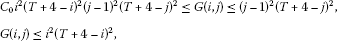where${C}_{0}=\frac{1}{3{\left(T+4\right)}^{7}}$.

For our constructions, we shall consider the Banach space $E=C\left(\left[0,T+4\right]\right)$ equipped with the standard norm $\parallel x\parallel ={max}_{0\le i\le T+4}|x\left(i\right)|$, $x\in E$. We define a cone $P\subset E$ by

$P=\left\{x\in E|x\left(i\right)\ge {C}_{0}{i}^{2}{\left(T+4-i\right)}^{2}\parallel x\parallel ,i\in \left[0,T+4\right]\right\}.$

Define an operator $A\left(u,v\right)=\left({A}_{1}\left(u\right),{A}_{2}\left(v\right)\right):P×P\to E×E$ by

$\begin{array}{r}{A}_{1}u\left(i\right)=\sum _{j=2}^{T+2}G\left(i,j\right)\left({f}_{1}\left(i,v\left(i\right)\right)+{e}_{1}\left(i\right)\right),\phantom{\rule{1em}{0ex}}i\in \left[0,T+4\right],v\in P,\\ {A}_{2}v\left(i\right)=\sum _{j=2}^{T+2}G\left(i,j\right)\left({f}_{2}\left(i,u\left(i\right)\right)+{e}_{2}\left(i\right)\right),\phantom{\rule{1em}{0ex}}i\in \left[0,T+4\right],u\in P.\end{array}$
(5)

Notice from (5) and Lemma 2 that, for $u\in P$, we have

$\begin{array}{r}{A}_{1}u\left(i\right)=\sum _{j=2}^{T+2}G\left(i,j\right)\left({f}_{1}\left(i,v\left(i\right)\right)+{e}_{1}\left(i\right)\right)\le \sum _{j=2}^{T+2}{\left(j-1\right)}^{2}{\left(T+4-j\right)}^{2}\left({f}_{1}\left(i,v\left(i\right)\right)+{e}_{1}\left(i\right)\right),\\ {A}_{2}v\left(i\right)=\sum _{j=2}^{T+2}G\left(i,j\right)\left({f}_{2}\left(i,u\left(i\right)\right)+{e}_{2}\left(i\right)\right)\le \sum _{j=2}^{T+2}{\left(j-1\right)}^{2}{\left(T+4-j\right)}^{2}\left({f}_{2}\left(i,u\left(i\right)\right)+{e}_{2}\left(i\right)\right),\end{array}$

then $\parallel {A}_{1}v\parallel \le {\sum }_{j=2}^{T+2}{\left(j-1\right)}^{2}{\left(T+4-j\right)}^{2}\left({f}_{1}\left(i,v\left(i\right)\right)+{e}_{1}\left(i\right)\right)$ and $\parallel {A}_{2}u\parallel \le {\sum }_{j=2}^{T+2}{\left(j-1\right)}^{2}{\left(T+4-j\right)}^{2}\left({f}_{2}\left(i,u\left(i\right)\right)+{e}_{2}\left(i\right)\right)$.

On the other hand, we have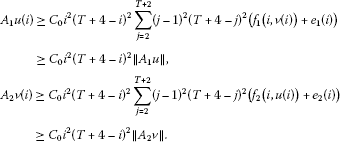Thus, $A\left(P×P\right)\subset P×P$. In addition, standard arguments show that A is completely continuous.

Now, we define the function ${\gamma }_{k}:E\to E$ by

${\gamma }_{k}\left(i\right)=\sum _{j=2}^{T+2}\frac{G\left(i,j\right)}{{i}^{2}{\left(T+4-i\right)}^{2}}{e}_{k}\left(j\right),\phantom{\rule{1em}{0ex}}i\in \left[0,T+4\right],k=1,2,$

which is the unique solution of

$\left\{\begin{array}{c}{\mathrm{△}}^{4}x\left(i-2\right)={e}_{k}\left(i\right),\phantom{\rule{1em}{0ex}}i\in \left[2,T+2\right],\hfill \\ x\left(0\right)=x\left(1\right)=x\left(T+3\right)=x\left(T+4\right)=0.\hfill \end{array}$

${\gamma }_{k\ast }=\underset{k,i}{min}{\gamma }_{k}\left(i\right),\phantom{\rule{2em}{0ex}}{\gamma }_{k}^{\ast }=\underset{k,i}{max}{\gamma }_{k}\left(i\right).$

## The case ${\gamma }_{1\ast }\ge 0$, ${\gamma }_{2\ast }\ge 0$

Theorem 1 Assume that there exists${b}_{k}\succ 0$, ${\stackrel{ˆ}{b}}_{k}\succ 0$and$0<{\alpha }_{k}<1$such that

$0\le \frac{{\stackrel{ˆ}{b}}_{k}\left(i\right)}{{x}^{{\alpha }_{k}}}\le {f}_{k}\left(i,x\right)\le \frac{{b}_{k}\left(i\right)}{{x}^{{\alpha }_{k}}},\phantom{\rule{1em}{0ex}}\mathit{\text{for all}}\phantom{\rule{0.25em}{0ex}}x>0,i\in \left[2,T+2\right],k=1,2.$
(H)

If${\gamma }_{1\ast }\ge 0$, ${\gamma }_{2\ast }\ge 0$, then there exists a positive solution of (1).

Proof A solution of (1) is just a fixed point of the completely continuous map $A\left(x,y\right)=\left({A}_{1}x,{A}_{2}y\right):P×P\to P×P$, from (5) we have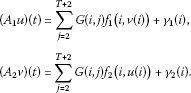By a direct application of Schauder’s fixed point theorem, the proof is finished if we prove that A maps the closed convex set defined as

$\begin{array}{rcl}K& =& \left\{\left(u,v\right)\in P×P:{r}_{1}{i}^{2}{\left(T+4-i\right)}^{2}\le u\left(i\right)\le {R}_{1}{i}^{2}{\left(T+4-i\right)}^{2},\\ {r}_{2}{i}^{2}{\left(T+4-i\right)}^{2}\le v\left(i\right)\le {R}_{2}{i}^{2}{\left(T+4-i\right)}^{2},i\in \left[0,T+4\right]\right\},\end{array}$

into itself, where ${R}_{1}>{r}_{1}>0$, ${R}_{2}>{r}_{2}>0$ are positive constants to be fixed properly. For convenience, we introduce the following notations

${\beta }_{k}\left(i\right)=\sum _{j=2}^{T+2}\frac{G\left(i,j\right)}{{i}^{2}{\left(T+4-i\right)}^{2}}{b}_{k}\left(j\right),\phantom{\rule{2em}{0ex}}{\stackrel{ˆ}{\beta }}_{k}\left(i\right)=\sum _{j=2}^{T+2}\frac{G\left(i,j\right)}{{i}^{2}{\left(T+4-i\right)}^{2}}{\stackrel{ˆ}{b}}_{k}\left(j\right),\phantom{\rule{1em}{0ex}}k=1,2.$

Given $\left(u,v\right)\in K$, by the nonnegative sign of G and ${f}_{k}$, $k=1,2$, we have

$\begin{array}{rl}\left({A}_{1}u\right)\left(i\right)& ={i}^{2}{\left(T+4-i\right)}^{2}\sum _{j=2}^{T+2}\frac{G\left(i,j\right)}{{i}^{2}{\left(T+4-i\right)}^{2}}{f}_{1}\left(j,v\left(j\right)\right)+{\gamma }_{1}\left(i\right)\\ \ge {i}^{2}{\left(T+4-i\right)}^{2}\sum _{j=2}^{T+2}\frac{G\left(i,j\right)}{{i}^{2}{\left(T+4-i\right)}^{2}}\frac{{\stackrel{ˆ}{b}}_{1}\left(j\right)}{{v}^{{\alpha }_{1}}\left(j\right)}+{i}^{2}{\left(T+4-i\right)}^{2}{\gamma }_{1\ast }\\ \ge {i}^{2}{\left(T+4-i\right)}^{2}\sum _{j=2}^{T+2}\frac{G\left(i,j\right)}{{i}^{2}{\left(T+4-i\right)}^{2}}\frac{{\stackrel{ˆ}{b}}_{1}\left(j\right)}{{R}_{2}^{{\alpha }_{1}}}\\ \ge {i}^{2}{\left(T+4-i\right)}^{2}{\stackrel{ˆ}{\beta }}_{1\ast }\cdot \frac{1}{{R}_{2}^{{\alpha }_{1}}}.\end{array}$

Note for every $\left(u,v\right)\in K$

$\begin{array}{rl}\left({A}_{1}u\right)\left(i\right)& ={i}^{2}{\left(T+4-i\right)}^{2}\sum _{j=2}^{T+2}\frac{G\left(i,j\right)}{{i}^{2}{\left(T+4-i\right)}^{2}}{f}_{1}\left(j,v\left(j\right)\right)+{\gamma }_{1}\left(i\right)\\ \le {i}^{2}{\left(T+4-i\right)}^{2}\sum _{j=2}^{T+2}\frac{G\left(i,j\right)}{{i}^{2}{\left(T+4-i\right)}^{2}}\frac{{b}_{1}\left(j\right)}{{v}^{{\alpha }_{1}}\left(j\right)}+{i}^{2}{\left(T+4-i\right)}^{2}{\gamma }_{1}^{\ast }\\ \le {i}^{2}{\left(T+4-i\right)}^{2}\sum _{j=2}^{T+2}\frac{G\left(i,j\right)}{{i}^{2}{\left(T+4-i\right)}^{2}}\frac{{b}_{1}\left(j\right)}{{r}_{2}^{{\alpha }_{1}}}+{i}^{2}{\left(T+4-i\right)}^{2}{\gamma }_{1}^{\ast }\\ \le {i}^{2}{\left(T+4-i\right)}^{2}\left({\beta }_{1}^{\ast }\cdot \frac{1}{{r}_{2}^{{\alpha }_{1}}}+{\gamma }_{1}^{\ast }\right).\end{array}$

Similarly, by the same strategy, we have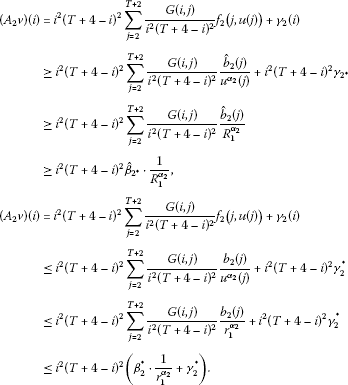Thus $\left({A}_{1}u,{A}_{2}v\right)\in K$ if ${r}_{1}$, ${r}_{2}$, ${R}_{1}$ and ${R}_{2}$ are chosen so that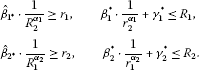Note that ${\stackrel{ˆ}{\beta }}_{i\ast },{\beta }_{i\ast }>0$ and taking $R={R}_{1}={R}_{2}$, $r={r}_{1}={r}_{2}$, $r=\frac{1}{R}$, it is sufficient to find $R>1$ such that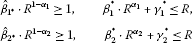and these inequalities hold for R big enough because ${\alpha }_{i}<1$. The proof is complete. □

## The case ${\gamma }_{1}^{\ast }\le 0$, ${\gamma }_{2}^{\ast }\le 0$

The aim of this section is to show that the presence of a weak singular nonlinearity makes it possible to find positive solutions if ${\gamma }_{1}^{\ast }\le 0$, ${\gamma }_{2}^{\ast }\le 0$.

Theorem 2 Assume that there exist${b}_{k},{\stackrel{ˆ}{b}}_{k}\succ 0$and$0<{\alpha }_{k}<1$, such that$\left(H\right)$is satisfied. If${\gamma }_{1}^{\ast }\le 0,{\gamma }_{2}^{\ast }\le 0$, and

$\begin{array}{r}{\gamma }_{1\ast }\ge {\left[{\alpha }_{1}{\alpha }_{2}\cdot \frac{{\stackrel{ˆ}{\beta }}_{1\ast }}{{\left({\beta }_{2}^{\ast }\right)}^{{\alpha }_{1}}}\right]}^{\frac{1}{1-{\alpha }_{1}{\alpha }_{2}}}\left(1-\frac{1}{{\alpha }_{1}{\alpha }_{2}}\right),\\ {\gamma }_{2\ast }\ge {\left[{\alpha }_{1}{\alpha }_{2}\cdot \frac{{\stackrel{ˆ}{\beta }}_{2\ast }}{{\left({\beta }_{1}^{\ast }\right)}^{{\alpha }_{2}}}\right]}^{\frac{1}{1-{\alpha }_{1}{\alpha }_{2}}}\left(1-\frac{1}{{\alpha }_{1}{\alpha }_{2}}\right),\end{array}$
(6)

then there exists a positive solution of (1).

Proof We follow the same strategy and notation as in the proof of ahead theorem. In this case, to prove that $A:K\to K$, it is sufficient to find $0<{r}_{1}<{R}_{1}$, $0<{r}_{2}<{R}_{2}$ such that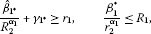(7)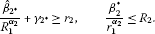(8)

If we fix ${R}_{1}=\frac{{\beta }_{1}^{\ast }}{{r}_{2}^{{\alpha }_{1}}}$, ${R}_{2}=\frac{{\beta }_{2}^{\ast }}{{r}_{1}^{{\alpha }_{2}}}$, then the first inequality of (8) holds if ${r}_{2}$ satisfies

${\stackrel{ˆ}{\beta }}_{2\ast }{\left({\beta }_{1}^{\ast }\right)}^{-{\alpha }_{2}}{r}_{2}^{{\alpha }_{1}{\alpha }_{2}}+{\gamma }_{2\ast }\ge {r}_{2},$

or equivalently

${\gamma }_{2\ast }\ge g\left({r}_{2}\right):={r}_{2}-\frac{{\stackrel{ˆ}{\beta }}_{2\ast }}{{\left({\beta }_{1}^{\ast }\right)}^{{\alpha }_{2}}}{r}_{2}^{{\alpha }_{1}{\alpha }_{2}}.$

The function $g\left({r}_{2}\right)$ possesses a minimum at

${r}_{20}:={\left[{\alpha }_{1}{\alpha }_{2}\cdot \frac{{\stackrel{ˆ}{\beta }}_{2\ast }}{{\left({\beta }_{1}^{\ast }\right)}^{{\alpha }_{2}}}\right]}^{\frac{1}{1-{\alpha }_{1}{\alpha }_{2}}}.$

Taking ${r}_{2}={r}_{20}$, then (6) holds if

${\gamma }_{2\ast }\ge g\left({r}_{20}\right)={\left[{\alpha }_{1}{\alpha }_{2}\cdot \frac{{\stackrel{ˆ}{\beta }}_{2\ast }}{{\left({\beta }_{1}^{\ast }\right)}^{{\alpha }_{2}}}\right]}^{\frac{1}{1-{\alpha }_{1}{\alpha }_{2}}}\left(1-\frac{1}{{\alpha }_{1}{\alpha }_{2}}\right).$

Similarly,

${\gamma }_{1\ast }\ge h\left({r}_{1}\right):={r}_{1}-\frac{{\stackrel{ˆ}{\beta }}_{1\ast }}{{\left({\beta }_{2}^{\ast }\right)}^{{\alpha }_{1}}}{r}_{1}^{{\alpha }_{1}{\alpha }_{2}},$

$h\left({r}_{1}\right)$ possesses a minimum at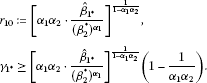Taking ${r}_{1}={r}_{10}$, ${r}_{2}={r}_{20}$, then the first inequalities in (7) and (8) hold if ${\gamma }_{1\ast }\ge g\left({r}_{1}\right)$ and ${\gamma }_{2\ast }\ge g\left({r}_{2}\right)$, which are just condition (6). The second inequalities hold directly from the choice of ${R}_{1}$ and ${R}_{2}$, so it remains to prove that ${R}_{1}=\frac{{\beta }_{1}^{\ast }}{{r}_{20}^{{\alpha }_{1}}}>{r}_{10}$, ${R}_{2}=\frac{{\beta }_{2}^{\ast }}{{r}_{10}^{{\alpha }_{2}}}>{r}_{20}$. This is easily verified through elementary computations:

$\begin{array}{rl}{R}_{1}& =\frac{{\beta }_{1}^{\ast }}{{r}_{20}^{{\alpha }_{1}}}=\frac{{\beta }_{1}^{\ast }}{{\left\{{\left[{\alpha }_{1}{\alpha }_{2}\cdot \frac{{\stackrel{ˆ}{\beta }}_{2\ast }}{{\left({\beta }_{1}^{\ast }\right)}^{{\alpha }_{2}}}\right]}^{\frac{1}{1-{\alpha }_{1}{\alpha }_{2}}}\right\}}^{{\alpha }_{1}}}\\ =\frac{{\beta }_{1}^{\ast }}{{\left[{\alpha }_{1}{\alpha }_{2}\cdot \frac{{\stackrel{ˆ}{\beta }}_{2\ast }}{{\left({\beta }_{1}^{\ast }\right)}^{{\alpha }_{2}}}\right]}^{\frac{{\alpha }_{1}}{1-{\alpha }_{1}{\alpha }_{2}}}}=\frac{{\left({\beta }_{1}^{\ast }\right)}^{1+\frac{{\alpha }_{1}{\alpha }_{2}}{1-{\alpha }_{1}{\alpha }_{2}}}}{{\left({\alpha }_{1}{\alpha }_{2}\cdot {\stackrel{ˆ}{\beta }}_{2\ast }\right)}^{\frac{{\alpha }_{1}}{1-{\alpha }_{1}{\alpha }_{2}}}}\\ =\frac{{\left({\beta }_{1}^{\ast }\right)}^{\frac{1}{1-{\alpha }_{1}{\alpha }_{2}}}}{{\left[{\left({\alpha }_{1}{\alpha }_{2}\cdot {\stackrel{ˆ}{\beta }}_{2\ast }\right)}^{{\alpha }_{1}}\right]}^{\frac{1}{1-{\alpha }_{1}{\alpha }_{2}}}}={\left[\frac{{\beta }_{1}^{\ast }}{{\left({\alpha }_{1}{\alpha }_{2}\cdot {\stackrel{ˆ}{\beta }}_{2\ast }\right)}^{{\alpha }_{1}}}\right]}^{\frac{1}{1-{\alpha }_{1}{\alpha }_{2}}}\\ ={\left[\frac{1}{{\left({\alpha }_{1}{\alpha }_{2}\right)}^{{\alpha }_{1}}}\cdot \frac{{\beta }_{1}^{\ast }}{{\left({\stackrel{ˆ}{\beta }}_{2\ast }\right)}^{{\alpha }_{1}}}\right]}^{\frac{1}{1-{\alpha }_{1}{\alpha }_{2}}}>{\left[{\alpha }_{1}{\alpha }_{2}\cdot \frac{{\stackrel{ˆ}{\beta }}_{1\ast }}{{\left({\beta }_{2}^{\ast }\right)}^{{\alpha }_{1}}}\right]}^{\frac{1}{1-{\alpha }_{1}{\alpha }_{2}}}={r}_{10},\end{array}$

since ${\stackrel{ˆ}{\beta }}_{k\ast }\le {\beta }_{k}^{\ast }$, $k=1,2$. Similarly, we have ${R}_{2}>{r}_{20}$. The proof is complete. □

## The case ${\gamma }_{1\ast }\ge 0$, ${\gamma }_{2}^{\ast }\le 0$ (${\gamma }_{1}^{\ast }\le 0$, ${\gamma }_{2\ast }\ge 0$)

Theorem 3 Assume (H) is satisfied. If${\gamma }_{1\ast }\ge 0$, ${\gamma }_{2}^{\ast }\le 0$and

${\gamma }_{2\ast }\ge {r}_{21}-{\stackrel{ˆ}{\beta }}_{2\ast }\cdot \frac{{r}_{21}^{{\alpha }_{1}{\alpha }_{2}}}{{\left({\beta }_{1}^{\ast }+{\gamma }_{1}^{\ast }{r}_{21}^{{\alpha }_{1}}\right)}^{{\alpha }_{2}}},$
(9)

where $0<{r}_{21}<+\mathrm{\infty }$ is a unique positive solution of the equation

${r}_{2}^{1-{\alpha }_{1}{\alpha }_{2}}{\left({\beta }_{1}^{\ast }+{\gamma }_{1}^{\ast }\cdot {r}_{2}^{{\alpha }_{1}}\right)}^{1+{\alpha }_{2}}={\alpha }_{1}{\alpha }_{2}{\beta }_{1}^{\ast }{\stackrel{ˆ}{\beta }}_{2\ast },$

then there exists a positive solution of (1).

Proof In this case,to prove that $A:K\to K$, it is sufficient to find ${r}_{1}<{R}_{1}$, ${r}_{2}<{R}_{2}$ such that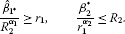(10)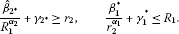(11)

If we fix ${R}_{2}=\frac{{\beta }_{2}^{\ast }}{{r}_{1}^{{\alpha }_{2}}}$, then the first inequality of (10) holds if ${r}_{1}$ satisfies

$\frac{{\stackrel{ˆ}{\beta }}_{1\ast }}{{\left({\beta }_{2}^{\ast }\right)}^{{\alpha }_{1}}}\cdot {r}_{1}^{{\alpha }_{1}{\alpha }_{2}}\ge {r}_{1},$

or equivalently

$0<{r}_{1}\le {\left[\frac{{\stackrel{ˆ}{\beta }}_{1\ast }}{{\left({\beta }_{2}^{\ast }\right)}^{{\alpha }_{1}}}\right]}^{\frac{1}{1-{\alpha }_{1}{\alpha }_{2}}}.$
(12)

If we chose ${r}_{1}>0$ small enough, then (12) holds, and ${R}_{2}$ is big enough.

If we fix ${R}_{1}=\frac{{\beta }_{1}^{\ast }}{{r}_{2}^{{\alpha }_{1}}}+{\gamma }_{1}^{\ast }$ then the first inequality of (11) holds if ${r}_{2}$ satisfies

$\begin{array}{rl}{\gamma }_{2\ast }& \ge {r}_{2}-\frac{{\stackrel{ˆ}{\beta }}_{2\ast }}{{R}_{1}^{{\alpha }_{2}}}\\ ={r}_{2}-{\stackrel{ˆ}{\beta }}_{2\ast }\cdot \frac{1}{{\left(\frac{{\beta }_{1}^{\ast }}{{r}_{2}^{{\alpha }_{1}}}+{\gamma }_{1}^{\ast }\right)}^{{\alpha }_{2}}}\\ ={r}_{2}-{\stackrel{ˆ}{\beta }}_{2\ast }\cdot \frac{1}{{\left(\frac{{\beta }_{1}^{\ast }+{\gamma }_{1}^{\ast }\cdot {r}_{2}^{{\alpha }_{1}}}{{r}_{2}^{{\alpha }_{1}}}\right)}^{{\alpha }_{2}}}\\ ={r}_{2}-{\stackrel{ˆ}{\beta }}_{2\ast }\cdot \frac{{r}_{2}^{{\alpha }_{1}{\alpha }_{2}}}{{\left({\beta }_{1}^{\ast }+{\gamma }_{1}^{\ast }\cdot {r}_{2}^{{\alpha }_{1}}\right)}^{{\alpha }_{2}}},\end{array}$

or equivalently

${\gamma }_{2\ast }\ge f\left({r}_{2}\right):={r}_{2}-{\stackrel{ˆ}{\beta }}_{2\ast }\cdot \frac{{r}_{2}^{{\alpha }_{1}{\alpha }_{2}}}{{\left({\beta }_{1}^{\ast }+{\gamma }_{1}^{\ast }\cdot {r}_{2}^{{\alpha }_{1}}\right)}^{{\alpha }_{2}}}.$

According to

$\begin{array}{rcl}{f}^{\prime }\left({r}_{2}\right)& =& 1-{\stackrel{ˆ}{\beta }}_{2\ast }\cdot \frac{1}{{\left({\beta }_{1}^{\ast }+{\gamma }_{1}^{\ast }\cdot {r}_{2}^{{\alpha }_{1}}\right)}^{2{\alpha }_{2}}}\cdot \left[{\alpha }_{1}{\alpha }_{2}{r}_{2}^{{\alpha }_{1}{\alpha }_{2}-1}{\left({\beta }_{1}^{\ast }+{\gamma }_{1}^{\ast }\cdot {r}_{2}^{{\alpha }_{1}}\right)}^{{\alpha }_{2}}\\ -{r}_{2}^{{\alpha }_{1}{\alpha }_{2}}{\alpha }_{2}{\left({\beta }_{1}^{\ast }+{\gamma }_{1}^{\ast }\cdot {r}_{2}^{{\alpha }_{1}}\right)}^{{\alpha }_{2}-1}{\alpha }_{1}{\gamma }_{1}^{\ast }{r}_{2}^{{\alpha }_{1}-1}\right]\\ =& 1-\frac{{\stackrel{ˆ}{\beta }}_{2\ast }{\alpha }_{1}{\alpha }_{2}{r}_{2}^{{\alpha }_{1}{\alpha }_{2}-1}}{{\left({\beta }_{1}^{\ast }+{\gamma }_{1}^{\ast }\cdot {r}_{2}^{{\alpha }_{1}}\right)}^{{\alpha }_{2}}}\left[1-\frac{{r}_{2}^{{\alpha }_{1}}{\gamma }_{1}^{\ast }}{{\beta }_{1}^{\ast }+{\gamma }_{1}^{\ast }\cdot {r}_{2}^{{\alpha }_{1}}}\right]\\ =& 1-{\alpha }_{1}{\alpha }_{2}{\beta }_{1}^{\ast }{\stackrel{ˆ}{\beta }}_{2\ast }{r}_{2}^{{\alpha }_{1}{\alpha }_{2}-1}{\left({\beta }_{1}^{\ast }+{\gamma }_{1}^{\ast }\cdot {r}_{2}^{{\alpha }_{1}}\right)}^{-1-{\alpha }_{2}},\end{array}$

we have ${f}^{\prime }\left(0\right)=-\mathrm{\infty }$, ${f}^{\prime }\left(+\mathrm{\infty }\right)=1$, then there exists ${r}_{21}$ such that ${f}^{\prime }\left({r}_{21}\right)=0$, and

$\begin{array}{rcl}{f}^{″}\left({r}_{2}\right)& =& -\left[{\alpha }_{1}{\alpha }_{2}{\beta }_{1}^{\ast }{\stackrel{ˆ}{\beta }}_{2\ast }\left({\alpha }_{1}{\alpha }_{2}-1\right){r}_{2}^{{\alpha }_{1}{\alpha }_{2}-2}{\left({\beta }_{1}^{\ast }+{\gamma }_{1}^{\ast }\cdot {r}_{2}^{{\alpha }_{1}}\right)}^{-1-{\alpha }_{2}}\\ +{\alpha }_{1}{\alpha }_{2}{\beta }_{1}^{\ast }{\stackrel{ˆ}{\beta }}_{2\ast }{r}_{2}^{{\alpha }_{1}{\alpha }_{2}-1}\left(-1-{\alpha }_{2}\right){\left({\beta }_{1}^{\ast }+{\gamma }_{1}^{\ast }\cdot {r}_{2}^{{\alpha }_{1}}\right)}^{-2-{\alpha }_{2}}{\gamma }_{1}^{\ast }{\alpha }_{1}{r}_{2}^{{\alpha }_{1}-1}\right]>0.\end{array}$

Then the function $f\left({r}_{2}\right)$ possesses a minimum at ${r}_{21}$, i.e., $f\left({r}_{21}\right)={min}_{{r}_{2}ϵ\left(0,+\mathrm{\infty }\right)}f\left({r}_{2}\right)$.

Note ${f}^{\prime }\left({r}_{21}\right)=0$, then we have

$1-{\alpha }_{1}{\alpha }_{2}{\beta }_{1}^{\ast }{\stackrel{ˆ}{\beta }}_{2\ast }{r}_{21}^{{\alpha }_{1}{\alpha }_{2}-1}{\left({\beta }_{1}^{\ast }+{\gamma }_{1}^{\ast }\cdot {r}_{21}^{{\alpha }_{1}}\right)}^{-1-{\alpha }_{2}}=0,$

or equivalently,

${r}_{21}^{1-{\alpha }_{1}{\alpha }_{2}}{\left({\beta }_{1}^{\ast }+{\gamma }_{1}^{\ast }\cdot {r}_{21}^{{\alpha }_{1}}\right)}^{1+{\alpha }_{2}}={\alpha }_{1}{\alpha }_{2}{\beta }_{1}^{\ast }{\stackrel{ˆ}{\beta }}_{2\ast }.$

Taking ${r}_{2}={r}_{21}$, then the first inequality in (11) holds if ${\gamma }_{2\ast }\ge f\left({r}_{21}\right)$, which is just condition (9). The second inequality holds directly by the choice of ${R}_{2}$, and it would remain to prove that ${r}_{21}<{R}_{2}$ and ${r}_{10}<{R}_{1}$. These inequalities hold for ${R}_{2}$ big enough and ${r}_{1}$ small enough. The proof is complete. □

Similarly, we have the following theorem.

Theorem 4 Assume (H) are satisfied. If${\gamma }_{1}^{\ast }\le 0$, ${\gamma }_{2\ast }\ge 0$and

${\gamma }_{1\ast }\ge {r}_{11}-{\stackrel{ˆ}{\beta }}_{1\ast }\cdot \frac{{r}_{11}^{{\alpha }_{1}{\alpha }_{2}}}{{\left({\beta }_{2}^{\ast }+{\gamma }_{2}^{\ast }{r}_{11}^{{\alpha }_{2}}\right)}^{{\alpha }_{1}}},$

where $0<{r}_{11}<+\mathrm{\infty }$ is a unique positive solution of the equation

${r}_{1}^{1-{\alpha }_{1}{\alpha }_{2}}{\left({\beta }_{2}^{\ast }+{\gamma }_{2}^{\ast }\cdot {r}_{1}^{{\alpha }_{2}}\right)}^{1+{\alpha }_{1}}={\alpha }_{1}{\alpha }_{2}{\beta }_{2}^{\ast }{\stackrel{ˆ}{\beta }}_{1\ast },$

then there exists a positive solution of (1).

## The case ${\gamma }_{1\ast }<0<{\gamma }_{1}^{\ast }$, ${\gamma }_{2\ast }<0<{\gamma }_{2}^{\ast }$

Theorem 5 Assume (H) is satisfied. If${\gamma }_{1\ast }<0<{\gamma }_{1}^{\ast }$, ${\gamma }_{2\ast }<0<{\gamma }_{2}^{\ast }$and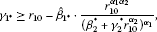(13)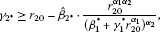(14)

where $0<{r}_{10}<+\mathrm{\infty }$ is a unique positive solution of the equation

${r}_{1}^{1-{\alpha }_{1}{\alpha }_{2}}{\left({\beta }_{2}^{\ast }+{\gamma }_{2}^{\ast }\cdot {r}_{1}^{{\alpha }_{2}}\right)}^{1+{\alpha }_{1}}={\alpha }_{1}{\alpha }_{2}{\beta }_{2}^{\ast }{\stackrel{ˆ}{\beta }}_{1\ast },$

and $0<{r}_{20}<+\mathrm{\infty }$ is a unique positive solution of the equation

${r}_{2}^{1-{\alpha }_{1}{\alpha }_{2}}{\left({\beta }_{1}^{\ast }+{\gamma }_{1}^{\ast }\cdot {r}_{2}^{{\alpha }_{1}}\right)}^{1+{\alpha }_{2}}={\alpha }_{1}{\alpha }_{2}{\beta }_{1}^{\ast }{\stackrel{ˆ}{\beta }}_{2\ast },$

then there exists a positive solution of (1).

Proof We follow the same strategy and notation as in the proof of ahead theorem. In this case, to prove that $A:K\to K$, it is sufficient to find ${r}_{1}<{R}_{1}$, ${r}_{2}<{R}_{2}$ such that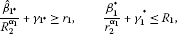(15)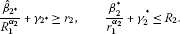(16)

If we fix ${R}_{1}=\frac{{\beta }_{1}^{\ast }}{{r}_{2}^{{\alpha }_{1}}}+{\gamma }_{1}^{\ast }$, ${R}_{2}=\frac{{\beta }_{2}^{\ast }}{{r}_{1}^{{\alpha }_{2}}}+{\gamma }_{2}^{\ast }$, then the first inequality of (15) holds if ${r}_{2}$ satisfies

${\gamma }_{2\ast }\ge g\left({r}_{2}\right):={r}_{2}-{\stackrel{ˆ}{\beta }}_{2\ast }\cdot \frac{{r}_{2}^{{\alpha }_{1}{\alpha }_{2}}}{{\left({\beta }_{1}^{\ast }+{\gamma }_{1}^{\ast }\cdot {r}_{2}^{{\alpha }_{1}}\right)}^{{\alpha }_{2}}}.$

According to

$\begin{array}{rcl}{g}^{\prime }\left({r}_{2}\right)& =& 1-{\stackrel{ˆ}{\beta }}_{2\ast }\cdot \frac{1}{{\left({\beta }_{1}^{\ast }+{\gamma }_{1}^{\ast }\cdot {r}_{2}^{{\alpha }_{1}}\right)}^{2{\alpha }_{2}}}\cdot \left[{\alpha }_{1}{\alpha }_{2}{r}_{2}^{{\alpha }_{1}{\alpha }_{2}-1}{\left({\beta }_{1}^{\ast }+{\gamma }_{1}^{\ast }\cdot {r}_{2}^{{\alpha }_{1}}\right)}^{{\alpha }_{2}}\\ -{r}_{2}^{{\alpha }_{1}{\alpha }_{2}}{\alpha }_{2}{\left({\beta }_{1}^{\ast }+{\gamma }_{1}^{\ast }\cdot {r}_{2}^{{\alpha }_{1}}\right)}^{{\alpha }_{2}-1}{\alpha }_{1}{\gamma }_{1}^{\ast }{r}_{2}^{{\alpha }_{1}-1}\right]\\ =& 1-\frac{{\stackrel{ˆ}{\beta }}_{2\ast }{\alpha }_{1}{\alpha }_{2}{r}_{2}^{{\alpha }_{1}{\alpha }_{2}-1}}{{\left({\beta }_{1}^{\ast }+{\gamma }_{1}^{\ast }\cdot {r}_{2}^{{\alpha }_{1}}\right)}^{{\alpha }_{2}}}\left[1-\frac{{r}_{2}^{{\alpha }_{1}}{\gamma }_{1}^{\ast }}{{\beta }_{1}^{\ast }+{\gamma }_{1}^{\ast }\cdot {r}_{2}^{{\alpha }_{1}}}\right]\\ =& 1-{\alpha }_{1}{\alpha }_{2}{\beta }_{1}^{\ast }{\stackrel{ˆ}{\beta }}_{2\ast }{r}_{2}^{{\alpha }_{1}{\alpha }_{2}-1}{\left({\beta }_{1}^{\ast }+{\gamma }_{1}^{\ast }\cdot {r}_{2}^{{\alpha }_{1}}\right)}^{-1-{\alpha }_{2}},\end{array}$

we have ${g}^{\prime }\left(0\right)=-\mathrm{\infty }$, ${g}^{\prime }\left(+\mathrm{\infty }\right)=1$, then there exists ${r}_{20}$ such that ${g}^{\prime }\left({r}_{20}\right)=0$, and

$\begin{array}{rcl}{g}^{″}\left({r}_{2}\right)& =& -\left[{\alpha }_{1}{\alpha }_{2}{\beta }_{1}^{\ast }{\stackrel{ˆ}{\beta }}_{2\ast }\left({\alpha }_{1}{\alpha }_{2}-1\right){r}_{2}^{{\alpha }_{1}{\alpha }_{2}-2}{\left({\beta }_{1}^{\ast }+{\gamma }_{1}^{\ast }\cdot {r}_{2}^{{\alpha }_{1}}\right)}^{-1-{\alpha }_{2}}\\ +{\alpha }_{1}{\alpha }_{2}{\beta }_{1}^{\ast }{\stackrel{ˆ}{\beta }}_{2\ast }{r}_{2}^{{\alpha }_{1}{\alpha }_{2}-1}\left(-1-{\alpha }_{2}\right){\left({\beta }_{1}^{\ast }+{\gamma }_{1}^{\ast }\cdot {r}_{2}^{{\alpha }_{1}}\right)}^{-2-{\alpha }_{2}}{\gamma }_{1}^{\ast }{\alpha }_{1}{r}_{2}^{{\alpha }_{1}-1}\right]>0.\end{array}$

Then the function $g\left({r}_{2}\right)$ possesses a minimum at ${r}_{20}$, i.e., $g\left({r}_{20}\right)={min}_{{r}_{2}ϵ\left(0,+\mathrm{\infty }\right)}g\left({r}_{2}\right)$.

Note ${g}^{\prime }\left({r}_{20}\right)=0$, then we have

$1-{\alpha }_{1}{\alpha }_{2}{\beta }_{1}^{\ast }{\stackrel{ˆ}{\beta }}_{2\ast }{r}_{21}^{{\alpha }_{1}{\alpha }_{2}-1}{\left({\beta }_{1}^{\ast }+{\gamma }_{1}^{\ast }\cdot {r}_{21}^{{\alpha }_{1}}\right)}^{-1-{\alpha }_{2}}=0,$

or equivalently,

${r}_{20}^{1-{\alpha }_{1}{\alpha }_{2}}{\left({\beta }_{1}^{\ast }+{\gamma }_{1}^{\ast }\cdot {r}_{20}^{{\alpha }_{1}}\right)}^{1+{\alpha }_{2}}={\alpha }_{1}{\alpha }_{2}{\beta }_{1}^{\ast }{\stackrel{ˆ}{\beta }}_{2\ast }.$

Similarly,

${\gamma }_{1\ast }\ge g\left({r}_{1}\right):={r}_{1}-{\stackrel{ˆ}{\beta }}_{1\ast }\cdot \frac{{r}_{1}^{{\alpha }_{1}{\alpha }_{2}}}{{\left({\beta }_{2}^{\ast }+{\gamma }_{2}^{\ast }\cdot {r}_{1}^{{\alpha }_{2}}\right)}^{{\alpha }_{1}}}.$

$g\left({r}_{10}\right)={min}_{{r}_{1}ϵ\left(0,+\mathrm{\infty }\right)}g\left({r}_{1}\right)$, and

${r}_{10}^{1-{\alpha }_{1}{\alpha }_{2}}{\left({\beta }_{2}^{\ast }+{\gamma }_{2}^{\ast }\cdot {r}_{10}^{{\alpha }_{2}}\right)}^{1+{\alpha }_{1}}={\alpha }_{1}{\alpha }_{2}{\beta }_{2}^{\ast }{\stackrel{ˆ}{\beta }}_{1\ast }.$

Taking ${r}_{1}={r}_{10}$ and ${r}_{2}={r}_{20}$, then the first inequality in (15) and (16) hold if ${\gamma }_{1\ast }\ge g\left({r}_{10}\right)$, ${\gamma }_{2\ast }\ge g\left({r}_{20}\right)$, which are just condition (13) and (14). The second inequalities hold directly by the choice of ${R}_{1}$ and ${R}_{2}$, and it would remain to prove that ${r}_{10}<{R}_{1}$ and ${r}_{20}<{R}_{2}$. This is easily verified through elementary computations.

$\begin{array}{rl}{R}_{1}& =\frac{{\beta }_{1}^{\ast }}{{r}_{20}^{{\alpha }_{1}}}+{\gamma }_{1}^{\ast }\\ =\frac{{\beta }_{1}^{\ast }+{\gamma }_{1}^{\ast }\cdot {r}_{20}^{{\alpha }_{1}}}{{r}_{20}^{{\alpha }_{1}}}\\ =\frac{{\left({\alpha }_{1}{\alpha }_{2}{\beta }_{1}^{\ast }{\stackrel{ˆ}{\beta }}_{2\ast }\right)}^{\frac{1}{1+{\alpha }_{2}}}\cdot {r}_{20}^{\frac{{\alpha }_{1}{\alpha }_{2}-1}{1+{\alpha }_{2}}}}{{r}_{20}^{{\alpha }_{1}}}\\ ={\left({\alpha }_{1}{\alpha }_{2}{\beta }_{1}^{\ast }{\stackrel{ˆ}{\beta }}_{2\ast }\right)}^{\frac{1}{1+{\alpha }_{2}}}\cdot {r}_{20}^{-\frac{1+{\alpha }_{1}}{1+{\alpha }_{2}}}.\end{array}$

The proof is the same as that in ${R}_{1}$, ${R}_{2}={\left({\alpha }_{1}{\alpha }_{2}{\beta }_{2}^{\ast }{\stackrel{ˆ}{\beta }}_{1\ast }\right)}^{\frac{1}{1+{\alpha }_{1}}}\cdot {r}_{10}^{-\frac{1+{\alpha }_{2}}{1+{\alpha }_{1}}}$.

Next, we will prove ${r}_{10}<{R}_{1}$, ${r}_{20}<{R}_{2}$, or equivalently,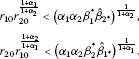Namely,

${r}_{10}^{1+{\alpha }_{2}}{r}_{20}^{1+{\alpha }_{1}}<{\alpha }_{1}{\alpha }_{2}{\beta }_{1}^{\ast }{\stackrel{ˆ}{\beta }}_{2\ast },{r}_{20}^{1+{\alpha }_{1}}{r}_{10}^{1+{\alpha }_{2}}<{\alpha }_{1}{\alpha }_{2}{\beta }_{2}^{\ast }{\stackrel{ˆ}{\beta }}_{1\ast }.$

On the other hand,

${r}_{20}^{1-{\alpha }_{1}{\alpha }_{2}}{\left({\beta }_{1}^{\ast }\right)}^{1+{\alpha }_{2}}\le {\alpha }_{1}{\alpha }_{2}{\beta }_{1}^{\ast }{\stackrel{ˆ}{\beta }}_{2\ast }.$

Then

${r}_{20}\le {\left({\alpha }_{1}{\alpha }_{2}{\left({\beta }_{1}^{\ast }\right)}^{-{\alpha }_{2}}{\stackrel{ˆ}{\beta }}_{2\ast }\right)}^{\frac{1}{1-{\alpha }_{1}{\alpha }_{2}}}.$

Similarly

${r}_{10}\le {\left({\alpha }_{1}{\alpha }_{2}{\left({\beta }_{2}^{\ast }\right)}^{-{\alpha }_{1}}{\stackrel{ˆ}{\beta }}_{1\ast }\right)}^{\frac{1}{1-{\alpha }_{1}{\alpha }_{2}}}.$

By (13) and (14),

${r}_{10}^{1+{\alpha }_{2}}{r}_{20}^{1+{\alpha }_{1}}\le {\left({\alpha }_{1}{\alpha }_{2}{\left({\beta }_{2}^{\ast }\right)}^{-{\alpha }_{1}}{\stackrel{ˆ}{\beta }}_{1\ast }\right)}^{\frac{1+{\alpha }_{2}}{1-{\alpha }_{1}{\alpha }_{2}}}{\left({\alpha }_{1}{\alpha }_{2}{\left({\beta }_{1}^{\ast }\right)}^{-{\alpha }_{2}}{\stackrel{ˆ}{\beta }}_{2\ast }\right)}^{\frac{1+{\alpha }_{1}}{1-{\alpha }_{1}{\alpha }_{2}}}.$

Now if we can prove

${\left({\alpha }_{1}{\alpha }_{2}{\left({\beta }_{2}^{\ast }\right)}^{-{\alpha }_{1}}{\stackrel{ˆ}{\beta }}_{1\ast }\right)}^{\frac{1+{\alpha }_{2}}{1-{\alpha }_{1}{\alpha }_{2}}}{\left({\alpha }_{1}{\alpha }_{2}{\left({\beta }_{1}^{\ast }\right)}^{-{\alpha }_{2}}{\stackrel{ˆ}{\beta }}_{2\ast }\right)}^{\frac{1+{\alpha }_{1}}{1-{\alpha }_{1}{\alpha }_{2}}}<{\alpha }_{1}{\alpha }_{2}{\beta }_{1}^{\ast }{\stackrel{ˆ}{\beta }}_{2\ast }$

then

${r}_{10}^{1+{\alpha }_{2}}{r}_{20}^{1+{\alpha }_{1}}<{\alpha }_{1}{\alpha }_{2}{\beta }_{1}^{\ast }{\stackrel{ˆ}{\beta }}_{2\ast }.$

In fact,

${\left({\alpha }_{1}{\alpha }_{2}\right)}^{\frac{2+{\alpha }_{2}+{\alpha }_{1}-1}{1-{\alpha }_{1}{\alpha }_{2}}}\cdot {\left(\frac{{\stackrel{ˆ}{\beta }}_{1\ast }}{{\beta }_{1}^{\ast }}\right)}^{\frac{1+{\alpha }_{2}}{1-{\alpha }_{1}{\alpha }_{2}}}\cdot {\left(\frac{{\stackrel{ˆ}{\beta }}_{2\ast }}{{\beta }_{2}^{\ast }}\right)}^{\frac{{\alpha }_{1}\left(1+{\alpha }_{2}\right)}{1-{\alpha }_{1}{\alpha }_{2}}}<1,$

since $\stackrel{ˆ}{\beta }i\ast \le {\beta }_{i}^{\ast }$, $i=1,2$. Similarly,we have ${r}_{20}^{1+{\alpha }_{1}}{r}_{10}^{1+{\alpha }_{2}}<{\alpha }_{1}{\alpha }_{2}{\beta }_{2}^{\ast }{\stackrel{ˆ}{\beta }}_{1\ast }$, we omit the details. Now we can obtain ${r}_{10}<{R}_{1}$, ${r}_{20}<{R}_{2}$. The proof is complete. □

## The case ${\gamma }_{1}^{\ast }\le 0$, ${\gamma }_{2\ast }<0<{\gamma }_{2}^{\ast }$ (${\gamma }_{2}^{\ast }\le 0$, ${\gamma }_{1\ast }<0<{\gamma }_{1}^{\ast }$)

Theorem 6 Assume (H) are satisfied. If${\gamma }_{1}^{\ast }\le 0$, ${\gamma }_{2\ast }<0<{\gamma }_{2}^{\ast }$and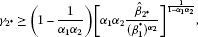(17)(18)

where $0<{r}_{11}<+\mathrm{\infty }$ is a unique positive solution of the equation

${r}_{1}^{1-{\alpha }_{1}{\alpha }_{2}}{\left({\beta }_{2}^{\ast }+{\gamma }_{2}^{\ast }\cdot {r}_{1}^{{\alpha }_{2}}\right)}^{1+{\alpha }_{1}}={\alpha }_{1}{\alpha }_{2}{\beta }_{2}^{\ast }{\stackrel{ˆ}{\beta }}_{1\ast },$
(19)

then there exists a positive solution of (1).

Proof In this case,to prove that $A:K\to K$, it is sufficient to find ${r}_{1}<{R}_{1}$, ${r}_{2}<{R}_{2}$ such that(20)(21)

If we fix ${R}_{1}=\frac{{\beta }_{1}^{\ast }}{{r}_{2}^{{\alpha }_{1}}}$, ${R}_{2}=\frac{{\beta }_{2}^{\ast }}{{r}_{1}^{{\alpha }_{2}}}+{\gamma }_{2}^{\ast }$, then the first inequality of (21) holds if ${r}_{2}$ satisfies

${\gamma }_{2\ast }\ge {r}_{2}-\frac{{\stackrel{ˆ}{\beta }}_{2\ast }}{{R}_{1}^{{\alpha }_{2}}}={r}_{2}-\frac{{\stackrel{ˆ}{\beta }}_{2\ast }}{{\left({\beta }_{1}^{\ast }\right)}^{{\alpha }_{2}}}\cdot {r}_{2}^{{\alpha }_{1}{\alpha }_{2}},$
(22)

or equivalently

${\gamma }_{2\ast }\ge f\left({r}_{2}\right):={r}_{2}-\frac{{\stackrel{ˆ}{\beta }}_{2\ast }}{{\left({\beta }_{1}^{\ast }\right)}^{{\alpha }_{2}}}\cdot {r}_{2}^{{\alpha }_{1}{\alpha }_{2}}.$
(23)

Then the function $f\left({r}_{2}\right)$ possesses a minimum at

${r}_{21}={\left[{\alpha }_{1}{\alpha }_{2}\cdot \frac{{\stackrel{ˆ}{\beta }}_{2\ast }}{{\left({\beta }_{1}^{\ast }\right)}^{{\alpha }_{2}}}\right]}^{\frac{1}{1-{\alpha }_{1}{\alpha }_{2}}},$
(24)

i.e., $f\left({r}_{21}\right)={min}_{{r}_{2}ϵ\left(0,+\mathrm{\infty }\right)}f\left({r}_{2}\right)$.

On the analogy of (22), we obtain

${\gamma }_{1\ast }\ge {r}_{1}-{\stackrel{ˆ}{\beta }}_{1\ast }\cdot \frac{{r}_{1}^{{\alpha }_{1}{\alpha }_{2}}}{{\left({\beta }_{2}^{\ast }+{\gamma }_{2}^{\ast }{r}_{1}^{{\alpha }_{2}}\right)}^{{\alpha }_{1}}},$
(25)

or equivalently,

${\gamma }_{1\ast }\ge h\left({r}_{1}\right):={r}_{1}-{\stackrel{ˆ}{\beta }}_{1\ast }\cdot \frac{{r}_{1}^{{\alpha }_{1}{\alpha }_{2}}}{{\left({\beta }_{2}^{\ast }+{\gamma }_{2}^{\ast }{r}_{1}^{{\alpha }_{2}}\right)}^{{\alpha }_{1}}}.$
(26)

According to

${h}^{\prime }\left({r}_{1}\right):=1-{\alpha }_{1}{\alpha }_{2}{\beta }_{2}^{\ast }{\stackrel{ˆ}{\beta }}_{1\ast }{r}_{1}^{{\alpha }_{1}{\alpha }_{2}-1}{\left({\beta }_{2}^{\ast }+{\gamma }_{2}^{\ast }{r}_{1}^{{\alpha }_{2}}\right)}^{-1-{\alpha }_{1}},$
(27)

we have ${h}^{\prime }\left(0\right)=-\mathrm{\infty }$, ${h}^{\prime }\left(+\mathrm{\infty }\right)=1$, then there exists ${r}_{11}$ such that ${h}^{\prime }\left({r}_{11}\right)=0$, and

$\begin{array}{rcl}{h}^{″}\left({r}_{1}\right)& =& -\left[{\alpha }_{1}{\alpha }_{2}{\beta }_{2}^{\ast }{\stackrel{ˆ}{\beta }}_{1\ast }\left({\alpha }_{1}{\alpha }_{2}-1\right){r}_{1}^{{\alpha }_{1}{\alpha }_{2}-2}{\left({\beta }_{2}^{\ast }+{\gamma }_{2}^{\ast }\cdot {r}_{1}^{{\alpha }_{2}}\right)}^{-1-{\alpha }_{1}}\\ +{\alpha }_{1}{\alpha }_{2}{\beta }_{2}^{\ast }{\stackrel{ˆ}{\beta }}_{1\ast }{r}_{1}^{{\alpha }_{1}{\alpha }_{2}-1}\left(-1-{\alpha }_{1}\right){\left({\beta }_{2}^{\ast }+{\gamma }_{2}^{\ast }\cdot {r}_{1}^{{\alpha }_{2}}\right)}^{-2-{\alpha }_{1}}{\gamma }_{2}^{\ast }{\alpha }_{2}{r}_{1}^{{\alpha }_{2}-1}\right]>0.\end{array}$
(28)

Then the function $h\left({r}_{1}\right)$ possesses a minimum at ${r}_{11}$, i.e., $h\left({r}_{11}\right)={min}_{{r}_{1}ϵ\left(0,+\mathrm{\infty }\right)}f\left({r}_{1}\right)$.

Note ${h}^{\prime }\left({r}_{11}\right)=0$, then we have

$1-{\alpha }_{1}{\alpha }_{2}{\beta }_{2}^{\ast }{\stackrel{ˆ}{\beta }}_{1\ast }{r}_{11}^{{\alpha }_{1}{\alpha }_{2}-1}{\left({\beta }_{2}^{\ast }+{\gamma }_{2}^{\ast }\cdot {r}_{11}^{{\alpha }_{2}}\right)}^{-1-{\alpha }_{1}}=0.$

Namely,

${r}_{11}^{1-{\alpha }_{1}{\alpha }_{2}}{\left({\beta }_{2}^{\ast }+{\gamma }_{2}^{\ast }\cdot {r}_{11}^{{\alpha }_{2}}\right)}^{1+{\alpha }_{1}}={\alpha }_{1}{\alpha }_{2}{\beta }_{2}^{\ast }{\stackrel{ˆ}{\beta }}_{1\ast }.$

Taking ${r}_{2}={r}_{21}$ and ${r}_{1}={r}_{11}$ then the first inequality in (20) and (21) hold if ${\gamma }_{2\ast }\ge h\left({r}_{21}\right)$ and ${\gamma }_{1\ast }\ge h\left({r}_{11}\right)$ which are just condition (17) and (18). The second inequalities hold directly by the choice of ${R}_{2}$ and ${R}_{1}$, so it would remain to prove that ${R}_{1}=\frac{{\beta }_{1}^{\ast }}{{r}_{21}^{{\alpha }_{1}}}>{r}_{11}$, ${R}_{2}=\frac{{\beta }_{2}^{\ast }}{{r}_{11}^{{\alpha }_{2}}}+{\gamma }_{2}^{\ast }>{r}_{21}$. Now we turn to prove that ${R}_{1}>{r}_{11}$, ${R}_{2}>{r}_{21}$.

First,

$\begin{array}{rl}{R}_{1}& =\frac{{\beta }_{1}^{\ast }}{{r}_{21}^{{\alpha }_{1}}}=\frac{{\beta }_{1}^{\ast }}{{\left\{{\left[{\alpha }_{1}{\alpha }_{2}\cdot \frac{{\stackrel{ˆ}{\beta }}_{2\ast }}{{\left({\beta }_{1}^{\ast }\right)}^{{\alpha }_{2}}}\right]}^{\frac{1}{1-{\alpha }_{1}{\alpha }_{2}}}\right\}}^{{\alpha }_{1}}}\\ =\frac{{\beta }_{1}^{\ast }}{{\left[{\alpha }_{1}{\alpha }_{2}\cdot \frac{{\stackrel{ˆ}{\beta }}_{2\ast }}{{\left({\beta }_{1}^{\ast }\right)}^{{\alpha }_{2}}}\right]}^{\frac{{\alpha }_{1}}{1-{\alpha }_{1}{\alpha }_{2}}}}=\frac{{\left({\beta }_{1}^{\ast }\right)}^{1+\frac{{\alpha }_{1}{\alpha }_{2}}{1-{\alpha }_{1}{\alpha }_{2}}}}{{\left({\alpha }_{1}{\alpha }_{2}\cdot {\stackrel{ˆ}{\beta }}_{2\ast }\right)}^{\frac{{\alpha }_{1}}{1-{\alpha }_{1}{\alpha }_{2}}}}\\ =\frac{{\left({\beta }_{1}^{\ast }\right)}^{\frac{1}{1-{\alpha }_{1}{\alpha }_{2}}}}{{\left[{\left({\alpha }_{1}{\alpha }_{2}\cdot {\stackrel{ˆ}{\beta }}_{2\ast }\right)}^{{\alpha }_{1}}\right]}^{\frac{1}{1-{\alpha }_{1}{\alpha }_{2}}}}={\left[\frac{{\beta }_{1}^{\ast }}{{\left({\alpha }_{1}{\alpha }_{2}\cdot {\stackrel{ˆ}{\beta }}_{2\ast }\right)}^{{\alpha }_{1}}}\right]}^{\frac{1}{1-{\alpha }_{1}{\alpha }_{2}}}\\ ={\left[\frac{1}{{\left({\alpha }_{1}{\alpha }_{2}\right)}^{{\alpha }_{1}}}\cdot \frac{{\beta }_{1}^{\ast }}{{\left({\stackrel{ˆ}{\beta }}_{2\ast }\right)}^{{\alpha }_{1}}}\right]}^{\frac{1}{1-{\alpha }_{1}{\alpha }_{2}}}>{\left[{\alpha }_{1}{\alpha }_{2}\cdot \frac{{\stackrel{ˆ}{\beta }}_{1\ast }}{{\left({\beta }_{2}^{\ast }\right)}^{{\alpha }_{1}}}\right]}^{\frac{1}{1-{\alpha }_{1}{\alpha }_{2}}}={r}_{11},\end{array}$

since ${\stackrel{ˆ}{\beta }}_{i\ast }\le {\beta }_{i}^{\ast }$, $i=1,2$.

On the other hand,

${R}_{2}=\frac{{\beta }_{2}^{\ast }}{{r}_{11}^{{\alpha }_{2}}}+{\gamma }_{2}^{\ast }=\frac{{\beta }_{2}^{\ast }+{\gamma }_{2}^{\ast }\cdot {r}_{11}^{{\alpha }_{2}}}{{r}_{11}^{{\alpha }_{2}}}.$
(29)

By (18), we have

${\beta }_{2}^{\ast }+{\gamma }_{2}^{\ast }\cdot {r}_{11}^{{\alpha }_{2}}={\left({\alpha }_{1}{\alpha }_{2}{\beta }_{2}^{\ast }{\stackrel{ˆ}{\beta }}_{1\ast }\right)}^{\frac{1}{1+{\alpha }_{1}}}{r}_{11}^{\frac{{\alpha }_{1}{\alpha }_{2}-1}{1+{\alpha }_{1}}}.$
(30)

Combing (29) and (30),

${R}_{2}={\left({\alpha }_{1}{\alpha }_{2}{\beta }_{2}^{\ast }{\stackrel{ˆ}{\beta }}_{1\ast }\right)}^{\frac{1}{1+{\alpha }_{1}}}{r}_{11}^{-\frac{1+{\alpha }_{2}}{1+{\alpha }_{1}}}.$
(31)

In what follows, we will verify that ${R}_{2}>{r}_{21}$. In fact,

${\left({\alpha }_{1}{\alpha }_{2}\right)}^{\frac{2+{\alpha }_{2}+{\alpha }_{1}}{1-{\alpha }_{1}{\alpha }_{2}}-1}\cdot {\left(\frac{{\stackrel{ˆ}{\beta }}_{2\ast }}{{\beta }_{2}^{\ast }}\right)}^{\frac{1+{\alpha }_{1}}{1-{\alpha }_{1}{\alpha }_{2}}}\cdot {\left(\frac{{\stackrel{ˆ}{\beta }}_{1\ast }}{{\beta }_{1}^{\ast }}\right)}^{\frac{{\alpha }_{2}\left(1+{\alpha }_{1}\right)}{1-{\alpha }_{1}{\alpha }_{2}}}<1,$

since ${\stackrel{ˆ}{\beta }}_{i\ast }\le {\beta }_{i}^{\ast }$, $i=1,2$. Thus

${\left({\alpha }_{1}{\alpha }_{2}{\beta }_{1}^{\ast \left(-{\alpha }_{2}\right)}{\stackrel{ˆ}{\beta }}_{2\ast }\right)}^{\frac{1+{\alpha }_{1}}{1-{\alpha }_{1}{\alpha }_{2}}}\cdot {\left({\alpha }_{1}{\alpha }_{2}{\beta }_{2}^{\ast \left(-{\alpha }_{1}\right)}{\stackrel{ˆ}{\beta }}_{1\ast }\right)}^{\frac{1+{\alpha }_{2}}{1-{\alpha }_{1}{\alpha }_{2}}}<{\alpha }_{1}{\alpha }_{2}{\beta }_{2}^{\ast }{\stackrel{ˆ}{\beta }}_{1\ast }.$
(32)

On the other hand,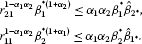Thus one can see easily note that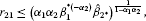(33)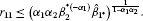(34)

From (33) and (34),

${r}_{11}^{1+{\alpha }_{2}}{r}_{21}^{1+{\alpha }_{1}}\le {\left({\alpha }_{1}{\alpha }_{2}{\beta }_{2}^{\ast \left(-{\alpha }_{1}\right)}{\stackrel{ˆ}{\beta }}_{1\ast }\right)}^{\frac{1+{\alpha }_{2}}{1-{\alpha }_{1}{\alpha }_{2}}}{\left({\alpha }_{1}{\alpha }_{2}{\beta }_{1}^{\ast \left(-{\alpha }_{2}\right)}{\stackrel{ˆ}{\beta }}_{2\ast }\right)}^{\frac{1+{\alpha }_{1}}{1-{\alpha }_{1}{\alpha }_{2}}}.$
(35)

Combing (32) and (35),

${r}_{11}^{1+{\alpha }_{2}}{r}_{21}^{1+{\alpha }_{1}}<{\alpha }_{1}{\alpha }_{2}{\beta }_{2}^{\ast }{\stackrel{ˆ}{\beta }}_{1\ast }.$

Therefore,

${r}_{21}{r}_{11}^{\frac{1+{\alpha }_{2}}{1+{\alpha }_{1}}}<{\left({\alpha }_{1}{\alpha }_{2}{\beta }_{2}^{\ast }{\stackrel{ˆ}{\beta }}_{1\ast }\right)}^{\frac{1}{1+{\alpha }_{1}}}.$

Recall (31), we obtain ${r}_{21}<{R}_{2}$ immediately. The proof is complete. □

Similarly, we have the following theorem.

Theorem 7 Assume (H) are satisfied. If${\gamma }_{2}^{\ast }\le 0$, ${\gamma }_{1\ast }<0<{\gamma }_{1}^{\ast }$and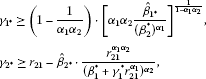where $0<{r}_{21}<+\mathrm{\infty }$ is a unique positive solution of the equation

${r}_{2}^{1-{\alpha }_{1}{\alpha }_{2}}{\left({\beta }_{1}^{\ast }+{\gamma }_{1}^{\ast }\cdot {r}_{2}^{{\alpha }_{1}}\right)}^{1+{\alpha }_{2}}={\alpha }_{1}{\alpha }_{2}{\beta }_{1}^{\ast }{\stackrel{ˆ}{\beta }}_{2\ast },$

then there exists a positive solution of (1).

## The case ${\gamma }_{1\ast }\ge 0$, ${\gamma }_{2\ast }<0<{\gamma }_{2}^{\ast }$ (${\gamma }_{2\ast }\ge 0$, ${\gamma }_{1\ast }<0<{\gamma }_{1}^{\ast }$)

Theorem 8 Assume (H) are satisfied. If${\gamma }_{1\ast }\ge 0$, ${\gamma }_{2\ast }<0<{\gamma }_{2}^{\ast }$and

${\gamma }_{2\ast }\ge {r}_{22}-{\stackrel{ˆ}{\beta }}_{2\ast }\cdot \frac{{r}_{22}^{{\alpha }_{1}{\alpha }_{2}}}{{\left({\beta }_{1}^{\ast }+{\gamma }_{1}^{\ast }{r}_{22}^{{\alpha }_{1}}\right)}^{{\alpha }_{2}}},$

where $0<{r}_{22}<+\mathrm{\infty }$ is a unique positive solution of the equation

${r}_{2}^{1-{\alpha }_{1}{\alpha }_{2}}{\left({\beta }_{1}^{\ast }+{\gamma }_{1}^{\ast }\cdot {r}_{2}^{{\alpha }_{1}}\right)}^{1+{\alpha }_{2}}={\alpha }_{1}{\alpha }_{2}{\beta }_{1}^{\ast }{\stackrel{ˆ}{\beta }}_{2\ast },$

then there exists a positive T-periodic solution of (1).

Proof In this case, to prove that $A:K\to K$, it is sufficient to find ${r}_{1}<{R}_{1}$, ${r}_{2}<{R}_{2}$ such that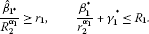(36)(37)

If we fix ${R}_{1}=\frac{{\beta }_{1}^{\ast }}{{r}_{2}^{{\alpha }_{1}}}+{\gamma }_{1}^{\ast }$, ${R}_{2}=\frac{{\beta }_{2}^{\ast }}{{r}_{1}^{{\alpha }_{2}}}+{\gamma }_{2}^{\ast }$, then the first inequality of (37) satisfies

${\stackrel{ˆ}{\beta }}_{2\ast }\cdot {\left(\frac{{\beta }_{1}^{\ast }}{{r}_{2}^{{\alpha }_{1}}}+{\gamma }_{1}^{\ast }\right)}^{-{\alpha }_{2}}+{\gamma }_{2\ast }\ge {r}_{2},$

or equivalently

${\gamma }_{2\ast }\ge l\left({r}_{2}\right):={r}_{2}-\frac{{\stackrel{ˆ}{\beta }}_{2\ast }}{{\left({\beta }_{1}^{\ast }+{\gamma }_{1}^{\ast }{r}_{2}^{{\alpha }_{1}}\right)}^{{\alpha }_{2}}}\cdot {r}_{2}^{{\alpha }_{1}{\alpha }_{2}}.$

Then the function $l\left({r}_{2}\right)$ possesses a minimum at ${r}_{22}$, i.e., $l\left({r}_{22}\right)={min}_{{r}_{2}ϵ\left(0,+\mathrm{\infty }\right)}l\left({r}_{2}\right)$.

Note ${l}^{\prime }\left({r}_{22}\right)=0$, then we have

$1-{\alpha }_{1}{\alpha }_{2}{\beta }_{1}^{\ast }{\stackrel{ˆ}{\beta }}_{2\ast }{r}_{22}^{{\alpha }_{1}{\alpha }_{2}-1}{\left({\beta }_{1}^{\ast }+{\gamma }_{1}^{\ast }\cdot {r}_{22}^{{\alpha }_{1}}\right)}^{-1-{\alpha }_{2}}=0.$

Therefore,

${r}_{22}^{1-{\alpha }_{1}{\alpha }_{2}}{\left({\beta }_{1}^{\ast }+{\gamma }_{1}^{\ast }\cdot {r}_{22}^{{\alpha }_{1}}\right)}^{1+{\alpha }_{2}}={\alpha }_{1}{\alpha }_{2}{\beta }_{1}^{\ast }{\stackrel{ˆ}{\beta }}_{2\ast }.$

Note that $\stackrel{ˆ}{\beta }i\ast ,{\beta }_{i\ast }>0$, $i=1,2$. And taking ${r}_{2}={r}_{22}$, ${R}_{1}=\frac{{\beta }_{1}^{\ast }}{{r}_{22}^{{\alpha }_{1}}}+{\gamma }_{1}^{\ast }$, ${r}_{1}=\frac{1}{{R}_{2}}$, it is sufficient to find ${r}_{1}<{R}_{1}$, ${r}_{2}<{R}_{2}$ such that

${R}_{2}^{{\alpha }_{1}-1}\le {\beta }_{1}^{\ast },\phantom{\rule{2em}{0ex}}{R}_{2}^{{\alpha }_{2}}{\beta }_{2}^{\ast }+{\gamma }_{2}^{\ast }\le {R}_{2}$

and these inequalities hold for ${R}_{2}$ big enough because ${\alpha }_{i}<1$. The proof is completed. □

Similarly, we have the following theorem.

Theorem 9 Assume (H) are satisfied. If${\gamma }_{2\ast }\ge 0$, ${\gamma }_{1\ast }<0<{\gamma }_{1}^{\ast }$and

${\gamma }_{1\ast }\ge {r}_{12}-{\stackrel{ˆ}{\beta }}_{1\ast }\cdot \frac{{r}_{12}^{{\alpha }_{1}{\alpha }_{2}}}{{\left({\beta }_{2}^{\ast }+{\gamma }_{2}^{\ast }{r}_{12}^{{\alpha }_{2}}\right)}^{{\alpha }_{1}}},$

where $0<{r}_{12}<+\mathrm{\infty }$ is a unique positive solution of the equation

${r}_{1}^{1-{\alpha }_{1}{\alpha }_{2}}{\left({\beta }_{2}^{\ast }+{\gamma }_{2}^{\ast }\cdot {r}_{1}^{{\alpha }_{2}}\right)}^{1+{\alpha }_{1}}={\alpha }_{1}{\alpha }_{2}{\beta }_{2}^{\ast }{\stackrel{ˆ}{\beta }}_{1\ast },$

then there exists a positive solution of (1).

## References

1. Agarwal RP: Difference Equations and Inequalities: Theory, Methods and Applications. Dekker, New York; 1992.

2. Agarwal RP, Henderson J: Positive solutions and nonlinear problems for third-order difference equation. Comput. Math. Appl. 1998, 36: 347–355. 10.1016/S0898-1221(98)80035-7

3. Agarwal RP, O’Regan D: Multiple solutions for higher-order difference equations. Comput. Math. Appl. 1999, 37: 39–48. 10.1016/S0898-1221(99)00112-1

4. Agarwal RP, O’Regan D: Discrete conjugate boundary value problems. Appl. Math. Lett. 1999, 37: 39–48.

5. Yuan C: Positive solutions of a singular positone and semipositone boundary value problems for fourth-order difference equations. Discrete Dyn. Nat. Soc. 2010., 2010: Article ID 312864

6. Avery RI, Chyan CJ, Henderson J: Twin solutions of boundary value problems for ordinary differential equations and finite difference equations. Comput. Math. Appl. 2001, 42: 695–704. 10.1016/S0898-1221(01)00188-2

7. Eloe PW: A generalization of concavity for finite differences. J. Math. Anal. Appl. 1998, 36(10–12):109–113.

8. Erbe LH, Xia HX, Yu JS: Global stability of linear non-autonomous delay difference equations. J. Differ. Equ. Appl. 1995, 1: 151–161. 10.1080/10236199508808016

9. Hao ZC: Nonnegative solutions for semilinear third-order difference equation boundary value problems. Acta Sci. Math. 2001, 21A: 225–229. in Chinese

10. Henderson J, Wong PJY: Positive solutions for a system of nonpositive difference equations. Aequ. Math. 2001, 62: 249–261. 10.1007/PL00000150

11. Kocic VL, Ladas G: Global Behavior of Nonlinear Difference Equations of Higher Order with Applications. Kluwer Academic, Boston; 1993.

12. Hatsunaga H, Hara T, Sakata S: Global attractivity for a nonlinear difference equation with variable delay. Comput. Math. Appl. 2001, 41: 543–551. 10.1016/S0898-1221(00)00297-2

13. Zhang B, Kong L, Sun Y, Deng X: Existence of positive solutions for BVPs of fourth-order difference equations. Appl. Math. Comput. 2002, 131: 583–591. 10.1016/S0096-3003(01)00171-0

14. Ma R, Gao C: Sign-changing solutions of nonlinear boundary value problems of difference equations. Indian J. Pure Appl. Math. 2008, 39: 323–332.

15. Bai D, Xu Y: Positive solutions for semipositone BVPs of second-order difference equations. Indian J. Pure Appl. Math. 2008, 39: 59–68.

16. He Z, Yu J: On the existence of positive solutions of fourth-order difference equations. Appl. Math. Comput. 2005, 161: 139–148. 10.1016/j.amc.2003.12.016

17. He T, Su Y: On discrete fourth-order boundary value problems with three parameters. J. Comput. Appl. Math. 2010, 233: 2506–2520. 10.1016/j.cam.2009.10.032

18. Yuan C, Jiang D, O’Regan D: Existence and uniqueness of positive solutions for fourth-order nonlinear singular continuous and discrete boundary value problems. Appl. Math. Comput. 2008, 203: 194–201. 10.1016/j.amc.2008.04.034

19. Jobe J, Mingay D: Cognition and survey measurement: history and overview. Appl. Cogn. Psychol. 1991, 5(3):175–192. 10.1002/acp.2350050303

20. Oppenheim AN: Questionnaire Design, Interviewing and Attitude Measurement. 2nd edition. Continuum International Publishing, New York; 1992.

21. Momani, SM: Some problems in non-Newtonian fluid mechanics. PhD thesis, Wales University, UK (1991)

22. Ma TF, Silva J: Iteration solution for a beam equation with nonlinear boundary conditions of third order. Appl. Math. Comput. 2004, 159: 11–18. 10.1016/j.amc.2003.08.088

23. Chawla MM, Katti CP: Finite difference methods for two-point boundary-value problems involving higher order differential equation. BIT Numer. Math. 1979, 19: 27–33. 10.1007/BF01931218

24. Pei M, Chang SK: Monotone iterative technique and symmetric positive solutions for a fourth-order boundary value problem. Math. Comput. Model. 2010, 51: 1260–1267. 10.1016/j.mcm.2010.01.009

25. Ma D, Yang X: Upper and lower solution method for fourth-order four-point boundary value problems. J. Comput. Appl. Math. 2009, 223: 543–551. 10.1016/j.cam.2007.10.051

26. Feng H, Ji D, Ge W: Existence and uniqueness of solutions for a fourth-order boundary value problem. Nonlinear Anal. 2009, 70: 3561–3566. 10.1016/j.na.2008.07.013

27. Ramadan MA, Lashien IF, Zahra WK: Quintic nonpolynomial spline solutions for fourth-order two-point boundary value problem. Commun. Nonlinear Sci. Numer. Simul. 2009, 14: 1105–1114. 10.1016/j.cnsns.2007.12.008

## Acknowledgement

This study was supported by the National Science Founds of China (NO. 71172019).

## Author information

Authors

### Corresponding author

Correspondence to Yupeng Fei.

### Competing interests

The authors declare that they have no competing interests.

### Authors’ contributions

WL, YF, BS, and YP have critically revised the manuscript and have made substantial contributions to conception. WL designed the research and drafted the manuscript. YF, BS and YP revised it critically for important intellectual content. All authors read and approved the final manuscript.

## Rights and permissions

Reprints and Permissions

Li, W., Fei, Y., Shan, B. et al. Positive solutions of a singular semipositone boundary value problems for fourth-order coupled difference equations. Adv Differ Equ 2012, 97 (2012). https://doi.org/10.1186/1687-1847-2012-97

• Accepted:

• Published:

• DOI: https://doi.org/10.1186/1687-1847-2012-97

### Keywords

• positive solution
• positone and semipositone boundary value problem
• singular difference equation
• fixed point theorem